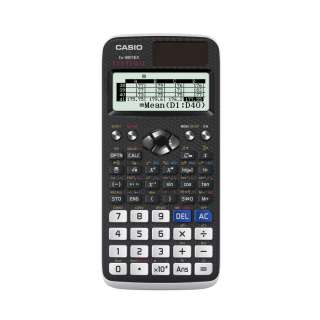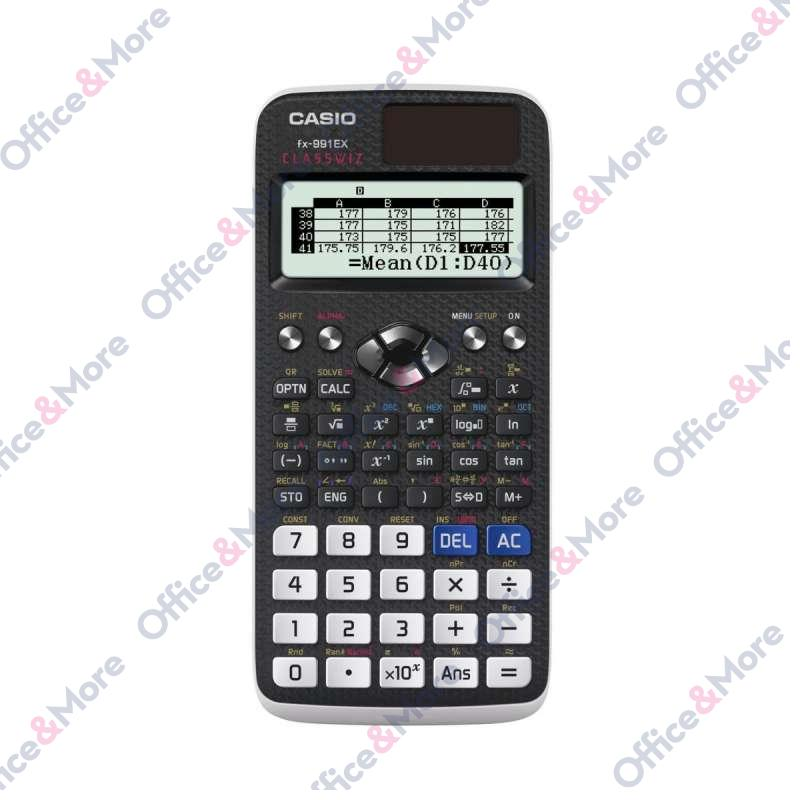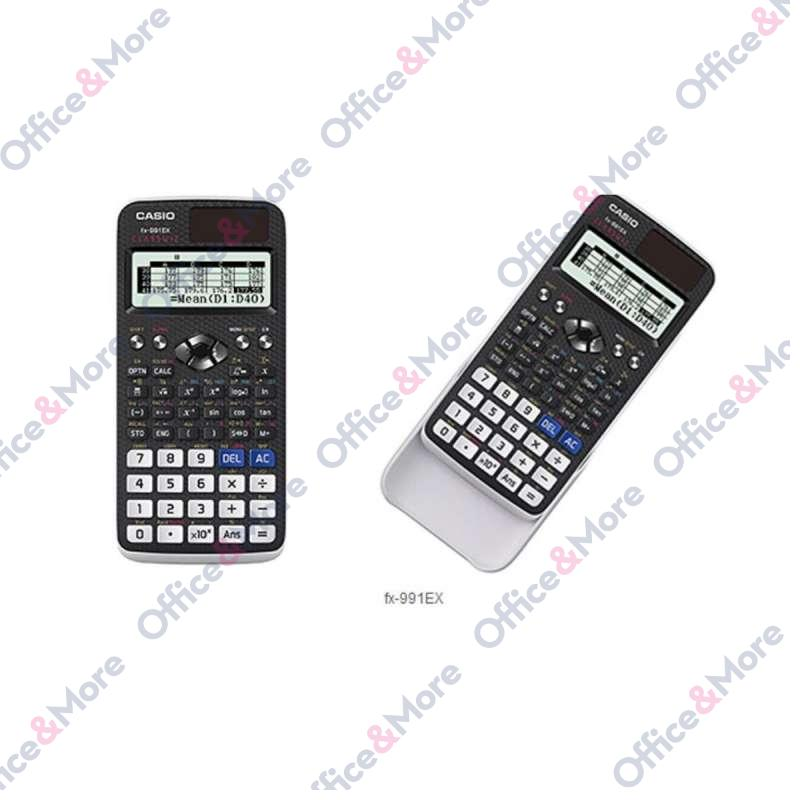CASIO

# CASIO KALKULATOR FX-991EX

Šifra artikla: 2501150103035
Proveri dostupnost
Sačuvajte u listi želja
Detailled Specification:

High-resolution LC display with 192 x 63 pixels
Natural-V.P.A.M. – Natural display
552 functions
QR code function¹
Solving equations and systems of up to four equations
4X4 matrices
Vector calculations
Integration calculations
Differential calculations
Probability distribution
Calculation with technical symbols
Undo-Function
Complex number calculation
Base-N calculations
Equation calculations
Inequality calculation
SUM calculation
47 wcientific constants
40 metrixc conversions
Automatic parenthesis
24 Parentheses level
Variable memory (9)
Trigonometric and invers trigonometric functions
Hyperbolic and invers hyperbolic functions
Power / Power Root calculations
logarithmic calculations
Exponential calculations
Root calculations
Combinatoric and Permutation
Prime factorization
Random Integer
Conversion of polar coordinates into rectangular coordinates and invers
Fraction (two modes)
Conversion of sexagesimal into decimal and invers
SCI/FIX/ENG function
List based statistics
1-variable statistics
Standard deviation
2-variable statistics (Regressions)
Percentage calculation
Non-natural input
Output with √ form
Output with Π form
Function table

General specifications

552 functions
Display: „Natural Textbook Display“ Natural V.P.A.M.
High-Resolution LC-Display, 63 x192 dots, 16/10 + 2 digits
Energy supply: Solar + Battery (1xLR44)
Comes with new slide-on hardcase
Dimensions (H x W x L): 77 x 165,5 x 11,1 mm
Weight: 90g
Karakteristika Vrednost
Kategorija Naučni kalkulatori
Robna Marka CASIO

### Ostavi komentar

Trenutno nema komentara